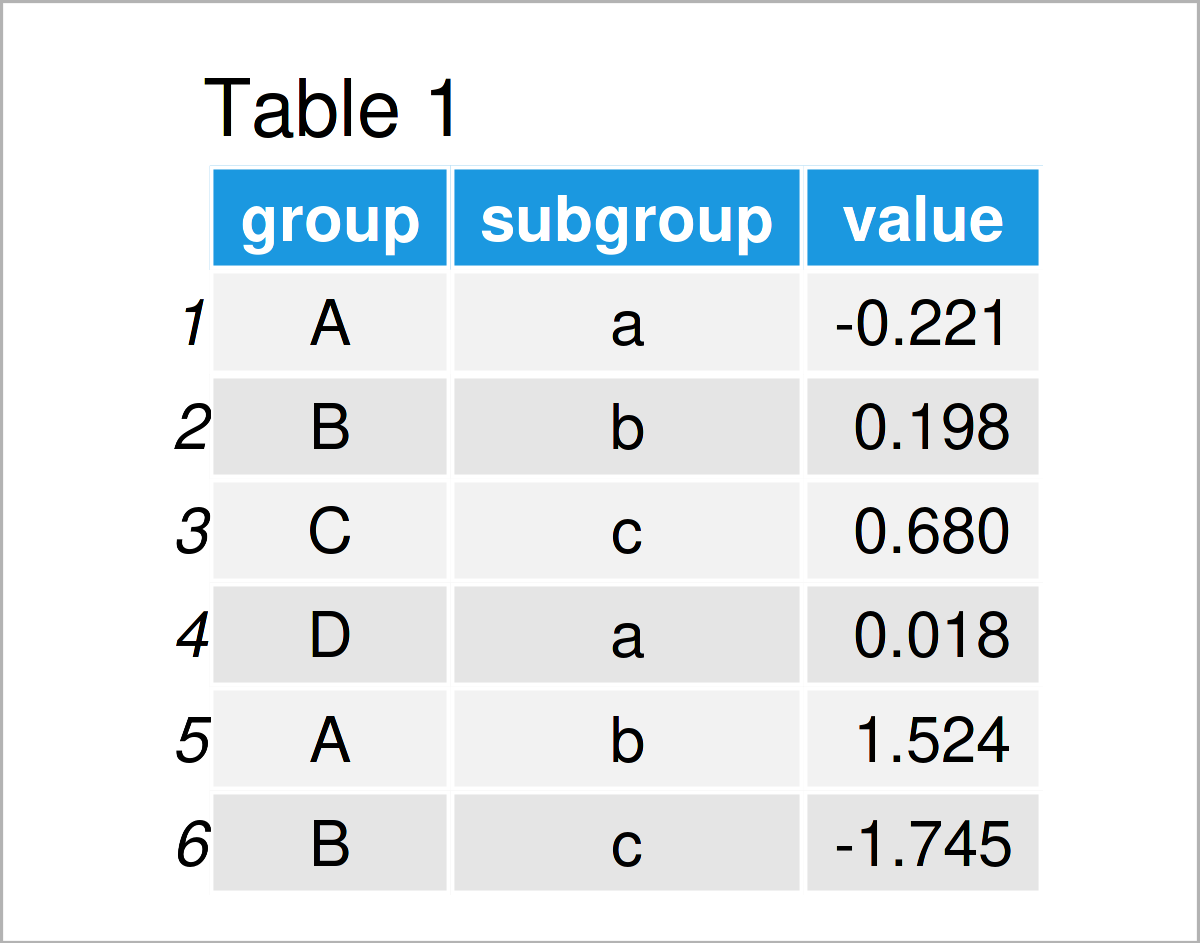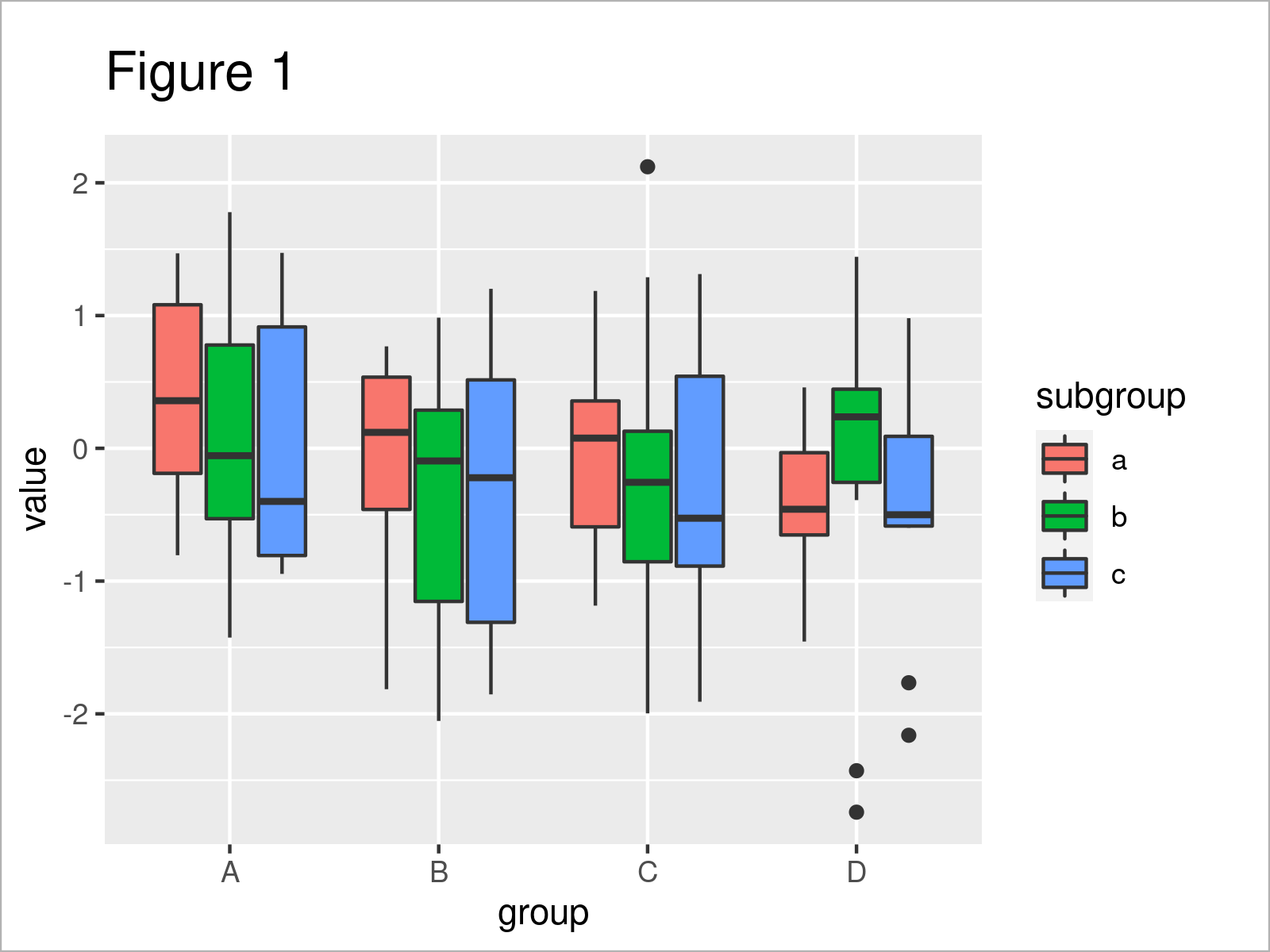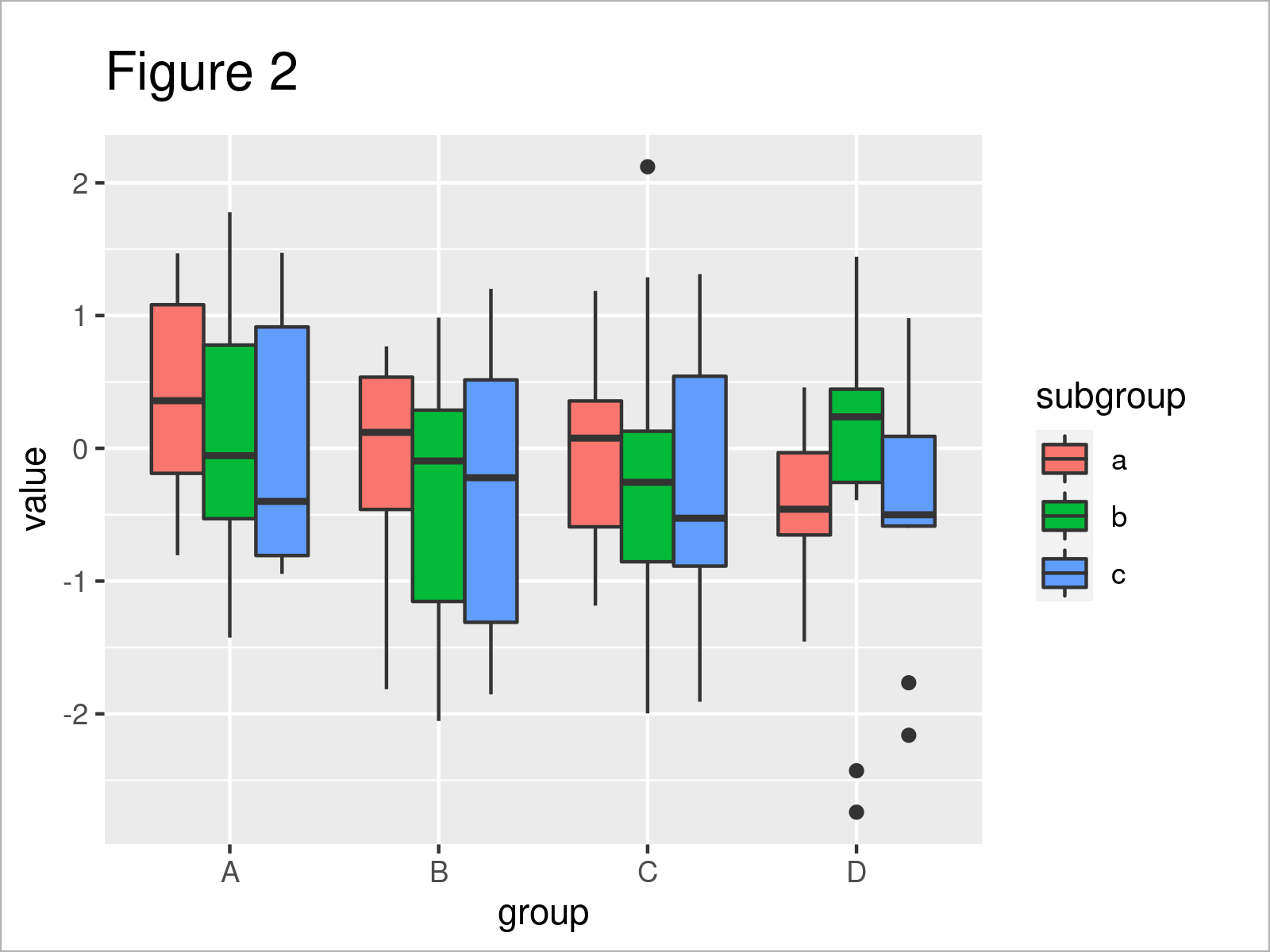# Modify Space Between Grouped ggplot2 Boxplots in R (2 Examples)

In this post you’ll learn how to adjust the spaces between boxplot groups in the R programming language.

Here’s the step-by-step process!

## Example Data, Packages & Basic Plot

The following data is used as basement for this R programming tutorial:

```set.seed(7859343)                     # Create example data
data <- data.frame(group = LETTERS[1:4],
subgroup = letters[1:3],
value = rnorm(120))As you can see based on Table 1, our exemplifying data is a data frame constituted of three variables. The variables group and subgroup have the character class and the variable value has the numeric class.

To be able to plot our example data with the ggplot2 package, we also have to install and load ggplot2:

```install.packages("ggplot2")           # Install & load ggplot2
library("ggplot2")```

Now, we can draw our data as follows.

```ggplot(data,                          # Boxplot with default spaces
aes(x = group,
y = value,
fill = subgroup)) +
geom_boxplot()```The output of the previous code is shown in Figure 1 – We have drawn a grouped boxplot with default spaces between the groups, i.e. smaller spaces as between the non-grouped boxplots.

## Example 1: Remove Space Between Grouped ggplot2 Boxplots

In Example 1, I’ll explain how to delete all space between ggplot2 boxplot groups.

For this, we have to set the position argument to be equal to “dodge” within the geom_boxplot function:

```ggplot(data,                          # Remove space between groups
aes(x = group,
y = value,
fill = subgroup)) +
geom_boxplot(position = "dodge")```As shown in Figure 2, the previous R programming syntax has created a grouped boxplot without any spaces between grouped boxes.

## Example 2: Equal Space Between All ggplot2 Boxplots

This example illustrates how to set all spaces to an equal width, no matter if the boxplots are grouped or not.

To achieve this, we have to set the position argument to be equal to position_dodge(1):

```ggplot(data,                          # Same space between all boxes
aes(x = group,
y = value,
fill = subgroup)) +
geom_boxplot(position = position_dodge(1))```Figure 3 shows the output of the previous R code – All spaces are arranged equally.

## Video, Further Resources & Summary

Some time ago I have released a video on my YouTube channel, which explains the examples of this article. You can find the video below:

In addition, you may want to read some of the related tutorials on my website. I have published several articles on topics such as graphics in R, labels, and ggplot2.

Summary: On this page you have learned how to modify the spaces between ggplot2 boxplot groups in R. Let me know in the comments below, in case you have additional questions. Furthermore, don’t forget to subscribe to my email newsletter for updates on the newest articles.

Subscribe to the Statistics Globe Newsletter